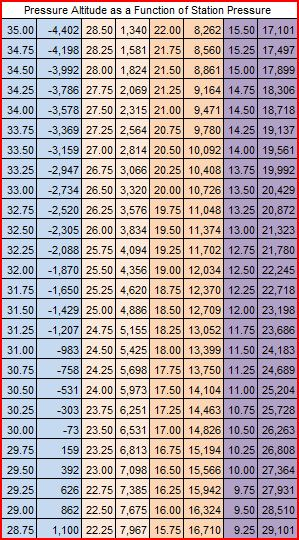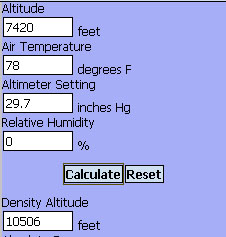## Manual Calculation of Density Altitude

by Linden B. (Lindy) Sisk

Last Revision December 3, 20101

Your Kestrel includes humidity in its calculation of density altitude. This method does not. However, it will get you pretty close if you don't have a Kestrel or other weather meter to do the calculation for you.

First we must calculate the pressure altitude, because barometric pressure varies, which affects the pressure altitude. The pressure altitude is the altitude an aircraft altimeter would read at our location if the reference pressure on the altimeter were set to the current sea-level barometric pressure.

Then we must compensate the pressure altitude for the temperature, which is usually different than the ICAO standard temperature at the pressure altitude.

The easiest method for doing this is to print copies of the table and the chart below, and use those.

### Step 1 Calculation of Pressure Altitude

If you have the station pressure from an instrument you carry, the easiest way to find the Pressure Altitude is to consult the table below. It's in increments of 0.25 inches of mercury. Just pick the nearest increment to your station pressure. If you aren't familiar with the distinction between station pressure and barometric pressure, you may wish to read this reference: Barometric Pressure and Ballistic SoftwareExample: The sensor in my Casio Pathfinder watch reads a pressure of 19.5 inches. Finding 19.5 in the table, I see the Pressure Altitude is 11,374 feet.

If you would like to download a copy of the Excel spreadsheet upon which the table above is based, so you can print your own copy, right-click on the following link: Pressure Altitude Spreadsheet.

If you have obtained the Pressure Altitude from the table above, you may skip to Step 2 below.

If we have no instrument to measure the local station pressure, but we know the sea-level barometric pressure, we can do this:

An approximation for the station pressure is:

Station Pressure = Sea-level Barometric Pressure - (Physical Altitude / 1000)

You may obtain the sea-level barometric pressure from the weather service. The sea-level barometric pressure is also what is reported by radio and TV stations.

Example: Your local TV station is reporting that the barometric pressure is 30.14 inches. You're at an altitude of 4,500 feet.

Station Pressure = 30.14 - (4500 / 1000) = 30.14 - 4.5 = 25.64

Then use the table above.

But what if we have nothing to tell us the atmospheric pressure at all? Then do this:

Pressure Altitude = 29.92 - (Physical Altitude / 1000)

No, it's not going to be precisely accurate, as it's based upon two assumptions, one that the barometric pressure at sea level is always 29.92, and a second that atmospheric pressure declines at the rate of 1 inch of mercury per thousand feet of altitude gain - but it's better than nothing.

### Step 2 Correction for Temperature

You can use this chart to do the temperature correction. Use the Pressure Altitude obtained from Step 1.

Find the blue line in the chart which corresponds to your pressure altitude, interpolating if necessary between the lines.

Then find the position on that line which corresponds to your temperature, using the scale at the bottom of the chart.

Read the Density Altitude in feet from the scale on the left side of the chart.Chart Courtesy of Michael Field

If you have obtained your Density Altitude from this chart, you may skip down to "How Accurate is this Calculation" below.

If you don't wish to use the chart, you may do the following steps: Use as an example a pressure altitude of 7,420 feet with a temperature of 78 degrees F. We have to correct that for the difference between the ICAO temperature and the actual temperature. Why do we have to do that? Hotter air is less dense than cooler air, so, if the air temperature is warmer than the ISA temperature at our altitude, the density altitude will be correspondingly higher.

#### Step 2a - Calculation of ISA Temperature at Pressure Altitude

ISA Temperature = 59 - (Pressure Altitude / 1000) * 3.6

ISA Temperature = 59 - (7420 / 1000) * 3.6

ISA Temperature = 59 - (7.42 * 3.6)

ISA Temperature = 59 - 26.712

ISA Temperature = 32.288, rounded to 32.29

#### Step 2b - Calculation of Temperature Correction in Feet

Correction (feet) = (Actual Temperature - ISA Temperature) * 66.67

Correction (feet) = (78 - 32.29) * 66.67

Correction (feet) = 3047 feet

#### Step 3 Add Pressure Altitude and Temperature Correction

Density Altitude = Pressure Altitude + Temperature Correction.

Density Altitude = 7420 + 3047

Density Altitude = 10467 feet

The increase from the temperature correction results from the fact that the temperature is above the ISA temperature for that altitude. If the temperature were below the ISA Temperature, the correction would be negative, and the Density Altitude would be lower than the pressure altitude.

### How Accurate is this Calculation?

If we put the data from the calculation above into the Density Altitude calculator here this is what we get:So, the error is not very large.

It's not as accurate as the calculation of density altitude from a Kestrel or other weather meter.

The calculation neglects humidity - but we know that humidity is a minor factor in shooting at distances out to about 1000 yards with most small arms capable of shooting to that distance with some accuracy.

If we are not using the station pressure to calculate the pressure altitude, we are also an making an assumption that the decline of barometric pressure with altitude is linear and about one inch of mercury per thousand feet. That isn't true at higher altitudes.

So, it's not perfectly accurate at longer distances at higher altitudes. The conclusion we might reasonably draw from that is, if you're going to use a Density Altitude Table for shooting long distances under differing environmental conditions, a Kestrel or other weather meter which calculates density altitude would be a useful piece of kit.

A method which gets us close to the correct answer is better than no answer at all.

Note 1: A precise formula for pressure altitude as a function of station pressure is here. I don't recommend it for manual calculation, unless you have access to a scientific calculator. If you're going to haul around a scientific calculator in the field, you might just as well haul a PDA with an installed ballistic program.

Revision Notes:

1. December 3, 2010. The only change was to correct a roundoff error in a calculation discovered by an alert reader. Thanks for the correction!